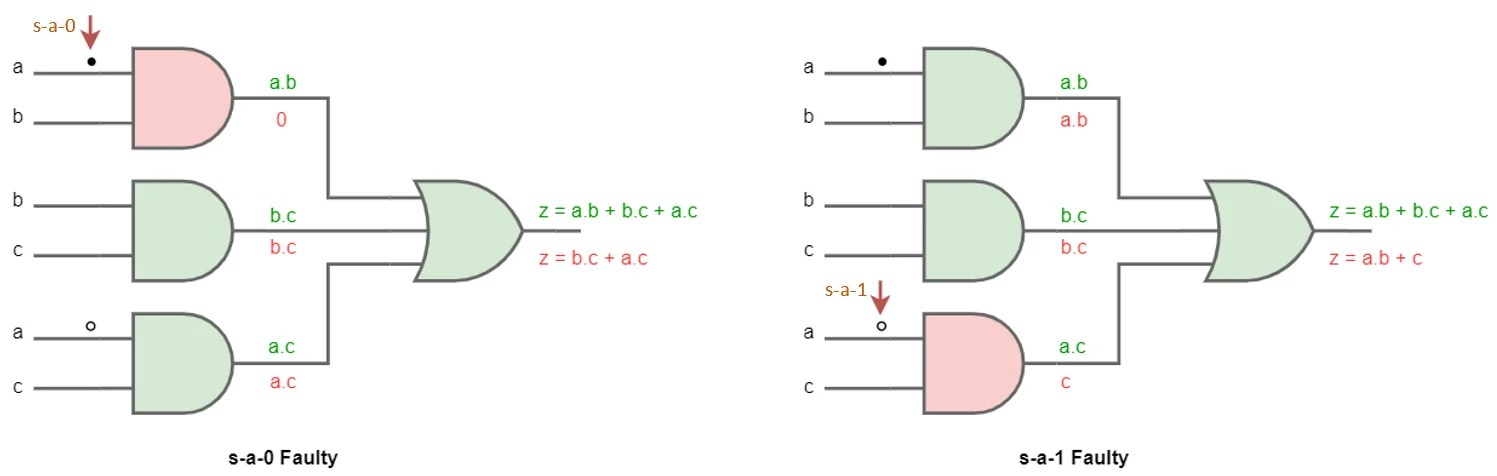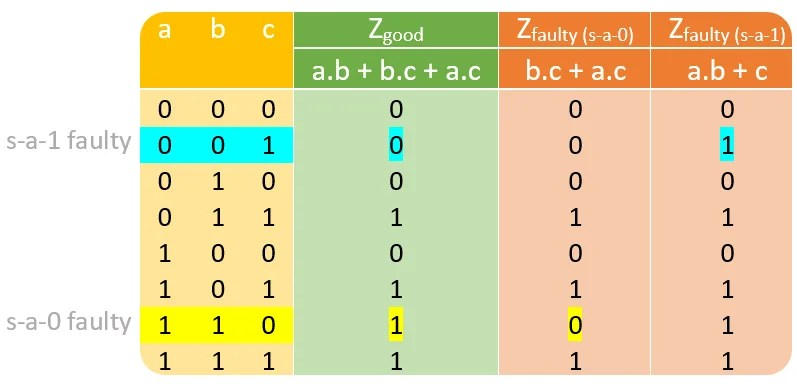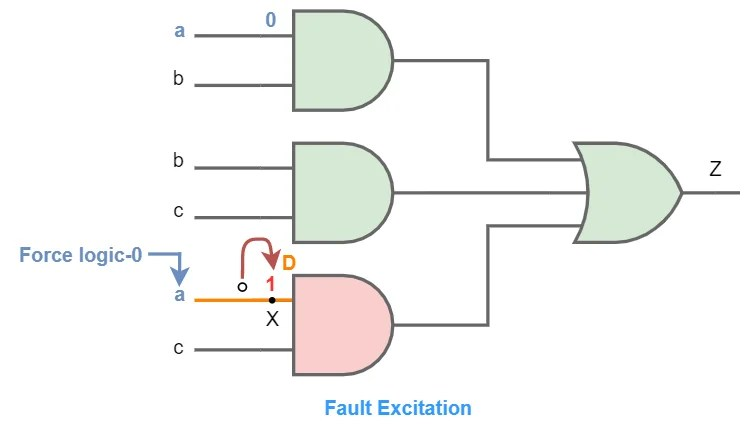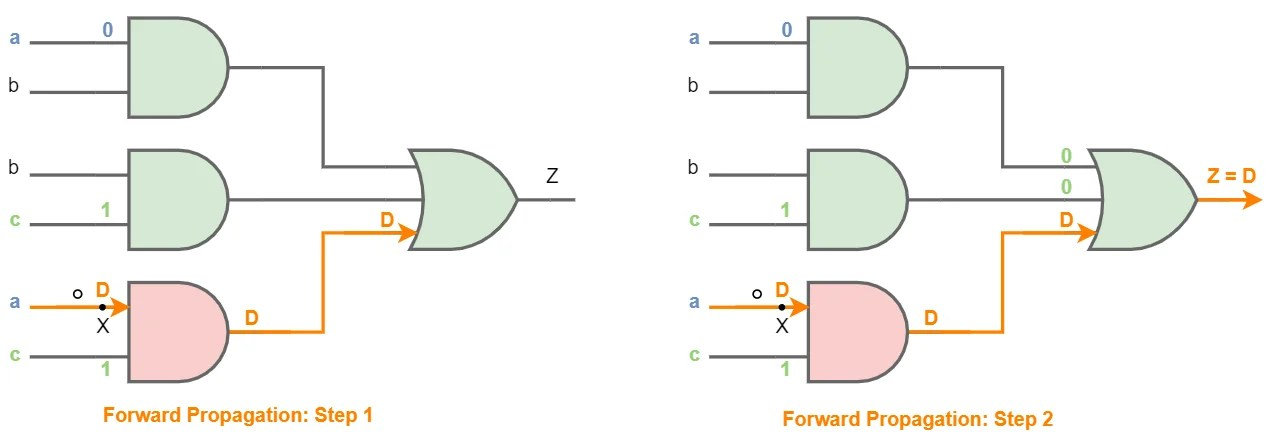View Course Path

# Test Generation Principles in DFT (VLSI)

Contents

## Test Generation or Fault Detection

In the previous post on fault modeling, we modeled common defects in circuits using faults at various levels of abstraction. We also generated test patterns to detect stuck-open/short faults in the Switch Level fault model. Interestingly we came to know that almost all other defects in other abstractions can be modeled as equivalent s-a-0/ s-a-1 faults. Due to this reason and simplicity, in this section (and also later on), we will primarily focus on the gate-level model.

The aim of test generation at the gate level is to verify that each logic gate in the circuit is functioning correctly, and the interconnections are good. If only a single stuck-at fault is assumed to be present in the circuit under test, then the problem is to construct a test set that will detect the fault by utilizing only the inputs and the outputs of the circuit.

Unlike the previous section, we can’t detect fault just by applying signals on any node in the circuit. Once the chip gets manufactured, the internal nodes are no more accessible. We only have control over the physical pins of the IC. Hence our job is to construct a stimulus to the circuit inputs, which will help us to detect the fault by observing the output result and comparing it with the desired result. The circuit which is being tested is also called CUT (Circuit Under Test).

Assuming a single stuck-at fault model, we apply a specific set of signals to the circuit input as a test. This is known as the Test Vector. A single test vector will only detect a particular stuck-at fault at a specific location. To detect all the stuck-at faults, we need to apply a series of test vectors sequentially. This series is also known as the Test Pattern.

Test Pattern= {Test Vector 1, Test Vector 2, Test Vector 3, …………………., Test Vector n}

## Why do we need test patterns?

Testing is not an easy process. Take an example of a combinational circuit IC, say 7483. It is a 4-bit full adder IC. It has a total of 14 logical pins out of which there are nine input pins (two 4-bit numbers and carry-in). The simplest way to test this chip is by verifying the truth-table. This can be done by applying each input combination and observing each corresponding output. There would be 29 = 512 total input combinations. So, it would require 512 steps or clock cycles to test this IC. This is also known as exhaustive testing.

Considering the operating frequency as 100 MHz, it would only take 512 / (100 x 106) = 5.12 µs to test. This is quite fast and feasible.

But this is a pretty dumb way of testing, because:

1. The test time increases exponentially with no. of input pins. The modern 64-bit microprocessor contains more than 500 input pins. Even for 50 input pins, the test time would be 250 / (100 x 106) = 130 days!
2. The chips which we use are mostly sequential circuits. In a sequential circuit, when we apply input, we cannot predict what the output will be because the output will depend on the state of the flip flops. If there are ‘S’ number of flip flops, there can be in 2S possible states. So, 2N possible inputs, coupled to 2S possible states, results in a complexity of 2N+S, making our job more difficult.
3. For the full-adder circuit, we can easily verify the results by looking into the output and comparing them. But most chips aren’t that simple. We may need a memory element or a Look-Up Table to store all the correct test results, which will be used in comparison during the testing process. This also increases the memory overhead exponentially with growing inputs.
Solutions to these problems?

These are the significant problems in the testing of the circuit. That’s why the subject DFT exists. All of these problems can be addressed using the following advanced DFT techniques, respectively.

1. Combinational ATPG
2. Sequential ATPG and structured techniques like Scan Path, Boundary Scan
3. BIST and test compression

Make sure to go through these sections in this free DFT course too.

## Test Generation Methods

It is noteworthy to notice that though it’s a time-consuming process, the fault coverage (parameter to check how many faults can be picked up) for exhaustive testing in the combinational circuit is 100% since it checks for every possible primary input. But if we want to test some specific faults at a particular location, exhaustive testing is not the way to go. In this section, we will go through a few basic test generation principles, which are great alternatives to exhaustive testing for single stuck-at faults.

### Truth table Method

The most straightforward method for generating tests for a particular fault is to compare the truth-table of the fault-free and the faulty circuits. Consider a two-level circuit shown in the figure. This circuit has two faults, one s-a-1, and another s-a-0. We will consider these two faults separately (using two different figures) according to a single stuck-at fault model.The faulty gates, as well as faulty output, are shown in red, while good ones are shown in green. The truth table for both the scenario can be formulated.  Always remember that • means stuck-at-0 fault and o means stuck-at-1.Observe that for most of the input stimuli, Zgood and Zfaulty give the same response. So, even the faulty circuit behaves properly most of the time. Note that we can only observe output Z to determine whether the circuit is faulty or not.

Hence, to detect the fault, we need to input a specific stimulus, which will result in different output responses for both Zgood and Zfaulty. That’s how we will be able to detect the fault by observing different values than expected. These are highlighted in yellow as well as blue for s-a-0 fault and s-a-1 fault respectively. For all the other cases, we can observe that the output response is the same.

Hence, the test vector for faulty (s-a-0) circuit is (a, b, c) = (1, 1, 0). While the test vector for faulty (s-a-1) circuit is (a, b, c) = (0, 0, 1).

The test pattern comprises of both these test vectors and is represented by, (a, b, c)= {(1, 1, 0), (0, 0, 1)}. We just reduced the test pattern to 2-vectors from 8-vector exhaustive testing.

### Analytical XOR Method

The former method was straightforward, but it may take a lot of time to draw the truth-table every time, and the chances of mistake is pretty high. We are grown up now; let’s move into a more sophisticated version of the previous method.

In the previous method, we observed that for the fault to be detected, the output response must be different for the good or faulty circuit. This condition can be related by following Boolean equation:

Zgood ⊕ Zfaulty  = 1

This is an XOR function, and we very well know that XOR gives logic-1 only when both of its input, i.e. Zgood and Zfaulty, are different. So, this equation is the compressed version of what we did in the previous method. Now let’s find the test pattern for the faulty (s-a-0) circuit.${ Z }_{ good }\oplus { Z }_{ faulty(s-a-0) }\quad =\quad (ab+bc+ac)\oplus (bc+ac)\\ \qquad \qquad \qquad \qquad \quad =\quad (ab+bc+ac)'(bc+ac)\quad +\quad (ab+bc+ac)((b'+c')(a'+c'))\\ \qquad \qquad \qquad \qquad \quad =\quad (ab+(b+a)c)'((b+a)c)\quad +\quad (ab+bc+ac)(a'b'+b'c'+c'a'+c')\\ \qquad \qquad \qquad \qquad \quad =\quad (ab)'((b+a)c)'((b+a)c)\quad +\quad (ab+bc+ac)(a'b'+c')\\ \qquad \qquad \qquad \qquad \quad =\quad (ab+bc+ac)(a'b'+c')\\ \qquad \qquad \qquad \qquad \quad =\quad abc'\quad =\quad 1$

To satisfy the equation, {a, b, c} must be {1, 1, 0}. This test vector is exactly similar to what we got in the truth-table method for faulty (s-a-0) circuit. Similarly, we can find the test set for the faulty (s-a-1) circuit too.

### Path Sensitization Method

The basic principle of the path sensitization method is to choose some path from the origin of the fault to the circuit output. A path is sensitized if inputs to the gates along the path are assigned values such that the effect of the fault can be propagated to the output.

Sensitization is a part of the test generation process in which appropriate stimulus is applied at the primary inputs so that the effect of the fault is observable at the primary outputs.

To illustrate, let us consider the s-a-1 faulty condition of the previous circuit. In the example, the effect of the stuck-at-1 fault can only be observed at output ‘Z’ if input ‘a’ is set logic-0. If ‘a’ is set to logic-1, there will be no difference in a faulty and good circuit; hence the effect of the fault will be masked. The path sensitization method is done in three steps:

1. Fault excitation/activation
2. Forward propagation or sensitization
3. Backward propagation or justification

#### Fault excitationSo, our first step would be to create a discrepancy at the wire ‘X.’ This is done by forcing the net by an opposite value signal to which it is stuck-at. Since ‘X’ is stuck-at-1, we need to force logic-0 to this net, which simply translates to setting ‘a as logic-0. This process is also known as fault excitation or activation. Note that the net will still be stuck-at-1, but it will not match with our output ‘Z’ corresponding to logic-0 value at ‘a,’ as the circuit will operate thinking that the net ‘X’ is assigned logic-1.

After the fault is excited, we assign the net to a variable ‘D’ (stands for discrepancy). This is just an annotation to simplify the next steps. The effect of this discrepancy may or may not be observable at the output, as the other inputs (b and c) may mask the fault in later stages. We don’t want that. So, our next step is to propagate ‘D’ to the primary output.

#### Forward PropagationIn fault propagation, we observe the input of each forthcoming gate in the path of ‘D’ and assign desired values to the other secondary inputs, which will propagate discrepancy to the primary output. The orange line is the forward path for ‘D.’ Since this is a 2-level combinational circuit, we need to do propagation two times. This process is also known as forward propagation or sensitization.

In the first step, to pass ‘D’ through the AND-gate, the other input ‘c’ must be set to logic-0. If it is made logic-1, the output of AND gate will always be logic-0 and independent of ‘D.’ Hence, the input is ‘c’ is set to logic-1. Similarly, in the second step, the OR gate requires all the inputs to be set zero.

#### Backward PropagationNow, the path has been sensitized successfully. But we still didn’t get the test pattern as the value of ‘b’ is unknown. To find the value of ‘b,’ we just need to traverse behind every gate and assign the desired value to the net until we reach the primary inputs. This process is shown in green lines. This is known as backward propagation or justification. We can now read the test vector obtained at primary inputs i.e. (a, b, c) = (0, 0, 1) which is exactly similar to the test vector obtained in the truth-table method for the s-a-1 faulty circuit.

## Conclusion

We learned a few basic methods of test generation. We observed that these techniques prove highly effective for testing a single stuck-at fault model in contrast to exhaustive testing, which is time-consuming and also having many other drawbacks. These test generation principles are the building blocks of advanced test generation algorithms like combinational ATPG (Automatic Test Pattern Generation).

But before moving into those test generation algorithms, in the next article, we will consider few properties of faults which will help in reducing the test patterns even before test generation!

Related courses for this will be up soon!

This site uses Akismet to reduce spam. Learn how your comment data is processed.

Top